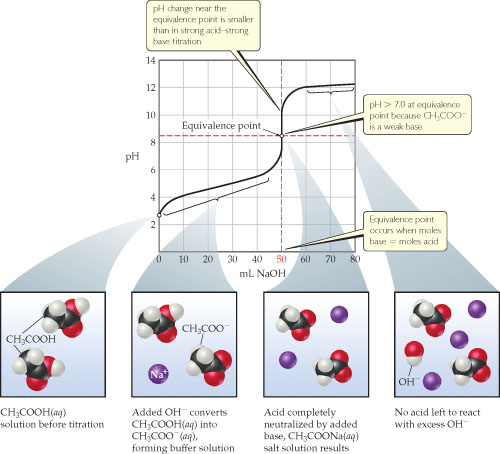# Problem: Titration of a weak acid with a strong base. The pH curve for titration of 50.0 mL of a 0.100 M solution of acetic acid with a 0.100 M solution of NaOH(aq). For clarity, water molecules have been omitted from the molecular art.If the acetic acid being titrated here were replaced by hydrochloric acid, would the amount of base needed to reach the equivalence point change?

###### FREE Expert Solution

We are asked if changing the acid from a weak acid to HCl would change the amount of NaOH need to reach the equivalence point.

CH3COOH is a weak acid and based on the Bronsted-Lowry definition, an acid is a proton (H+) donor. Once HClO loses its proton, its conjugate base is formed.

NaOH is a base and based on Bronsted-Lowry definition, a base is a proton (H+) acceptor.

CH3COOH will donate a proton to NaOH

Reaction:

CH3COOH (aq)    +   NaOH(aq)               COO(aq)       +       H2O(aq)
(weak acid)                     (base)                    (conjugate base)     (conjugate acid)

*Main group metals should have a +3 charge or higher to be considered acidic. Na+ has only a +1 charge and is a neutral ion so it will not contribute to the pH of the solution

97% (360 ratings)###### Problem DetailsTitration of a weak acid with a strong base. The pH curve for titration of 50.0 mL of a 0.100 M solution of acetic acid with a 0.100 M solution of NaOH(aq). For clarity, water molecules have been omitted from the molecular art.

If the acetic acid being titrated here were replaced by hydrochloric acid, would the amount of base needed to reach the equivalence point change?